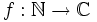Dirichlet series

Definition

Supposeis a function. In other words,is an arithmetic function. The Dirichlet series ofis defined as the following formal series:.

The corresponding function is defined as the sum of the series where convergence of the summation is considered in. Even though the series may converge on only a part of, it might be possible to extend to a global meromorphic function, or to a meromorphic function on a large part of.

We sometimes distinguish between the Dirichlet series (which is the formal series) and the Dirichlet function (which is the function obtained using the series and taking analytic continuation to parts of the complex plane where the series is not defined).